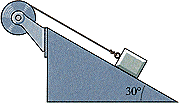# Rotational/Linear motion & friction

## Homework Statement

A 2.4 kg block rests on a 30° slope and is attached by a string of negligible mass to a solid drum of mass 0.80 kg and radius 5.0 cm, as shown in Fig. 10.29. When released, the block accelerates down the slope at 1.2 m/s2. What is the coefficient of friction between block and slope?

Ff=mu*N

a=alpha*r

Torque=I*alpha

## The Attempt at a Solution

Ok the first thing I did was draw a free-body diagram of the block and the drum. I have Fnet=mg-Tension-mu*Fnormal then and set Tension=torque and got T=1/2*Mdrum*a and put that back into the original equation and solved for mu, but I didn't get the right answer. Is this even the right way to do this?

tiny-tim
Homework Helper
Welcome to PF!

Hi Nick_L! Welcome to PF!A 2.4 kg block rests on a 30° slope and is attached by a string of negligible mass to a solid drum of mass 0.80 kg and radius 5.0 cm, as shown in Fig. 10.29. When released, the block accelerates down the slope at 1.2 m/s2. What is the coefficient of friction between block and slope?

I don't get it … what's the drum doing?is it rotating on a fixed horizontal axis, with the string unwinding, or on a fixed vertical axis, or is it sliding down the hill on its base?

Here is the picture associated with the problemtiny-tim
Homework Helper
ok … rotating on a fixed horizontal axis, presumably with a frictionless axle, and with the string unwinding …

so use the work-energy theorem … work done = energy lost, using the mass of the object and the moment of inertia of the drumOk so I would have Ffriction*dcos(30)=1/2*massbox*v2+1/2I*omega2? If that's right it makes sense, but how would I find displacement or velocity when I only have the acceleration?

tiny-tim
sorry, I missed the accelerationin that case, just use F = ma and torque = Iα and slug it out!Alright, thanks for the help